# Quantitative Aptitude Quiz for IBPS 2020 Mains Exams- 28th December

Directions (1-5): Each of the question below consists of a question and two statements numbered (i) and (ii) given below it. You have to decide whether the data provided in the statements is sufficient to answer the question. Read both the statements and give the answer.
(a) If the data in statement (I) alone is sufficient to answer the question, while the data in statement (II) alone is not sufficient to answer the question.
(b) If the data in statement (II) alone is sufficient to answer the question, while the data in statement (I) alone is not sufficient to answer the question.
(c) If the data either in statement (I) alone or in statement (II) alone is sufficient to answer the question.
(d) If the data both statements (I) and (II) together are not sufficient to answer the question.
(e) If the data in both statements (I) and (II) together are necessary to answer the question.

Q1. 6 men and 10 women are working together in a field. After working for 2 days, 3 men and 6 women leave the work. How many more days will be required to complete the work?
I. 12 men can complete three-fourth of the same work in 12 days
II. In 1 day, the work done by 2 men is equal to the work done by 3 women.

Q2. What percentage of simple interest per annum did Anuj pay to Aman?
I. Anuj borrowed Rs 5000 from Aman for 2 years
II. Anuj returned Rs 5500 to Aman at the end of 1st year and settled the loan.

Q3. What is the length of a running train crossing another 190-metre-long train running in the opposite direction?
I. The trains took 12 seconds to cross each other.
II. The relative speed of the two trains was 120 km/h

Q4. What is the profit percent gained by selling an almirah?
I. 4% discount is given on list price and if discount is not given, 25% profit is gained.
II. The cost price of the almirah is Rs 18,000.

Q5. Find the length of rectangle whose area is equal to the area of the right-angled triangle.
I. The base of the triangle is 30 cm
II. The height of the triangle is 60 cm.

Directions (6-10): In each of these questions, two equations (I) and (II) are given. You have to solve both the equations and give answer of the following questions: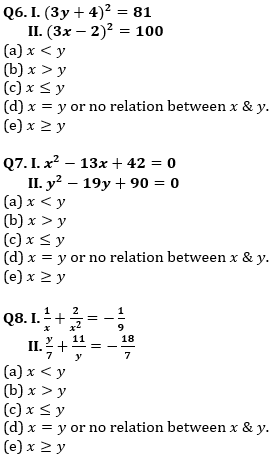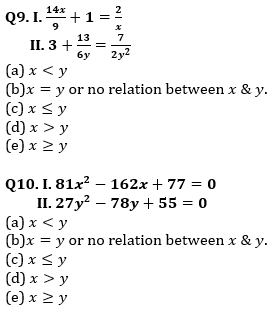Q11. A solid sphere is melted to form a toy with cylindrical base and conical top. Radius of sphere is 21cm and radius of toy is 33⅓% less than that of the sphere. Height of conical top of the toy is three times of the height of cylindrical base of the toy. Find height of the toy (in cm).
(a) 126
(b) 133
(c) 112
(d) 168
(e) 105

Q12. Veer keeps aside 10% of his monthly salary for saving and he spends his remaining salary on expenditure and investment (house rent, travel, clothes, etc.) and respective ratio of expenditure to investment is 5 : 3. If the amount spent on saving is Rs.7600 less than the amount spent by him on investments, then find Veer’s monthly salary?
(a) Rs.28000
(b) Rs.32000
(c) Rs.30000
(d) Rs.35000
(e) Rs.40000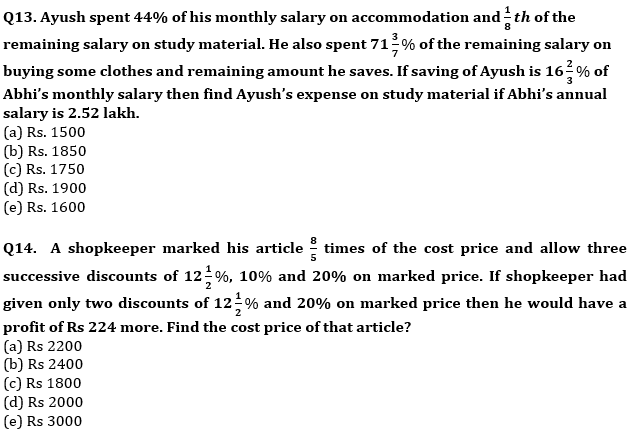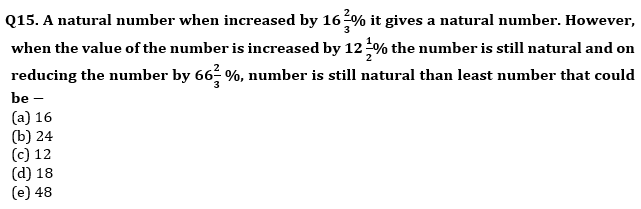Practice More Questions of Quantitative Aptitude for Competitive Exams:

###### Mains Quiz Study Plan for IBPS Exams 2020

Solutions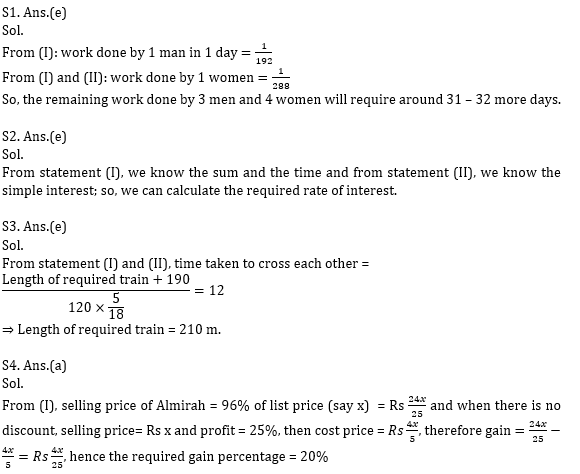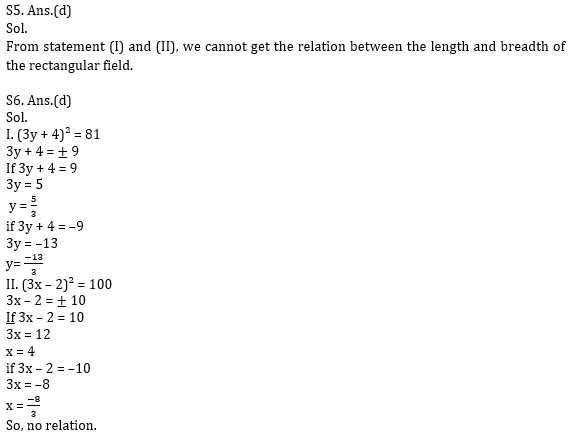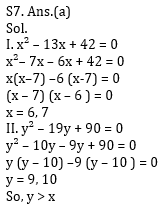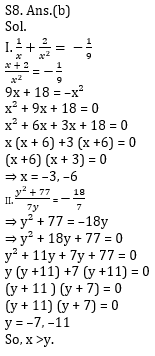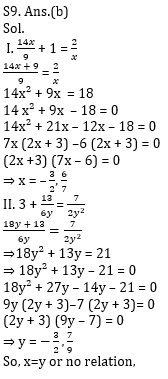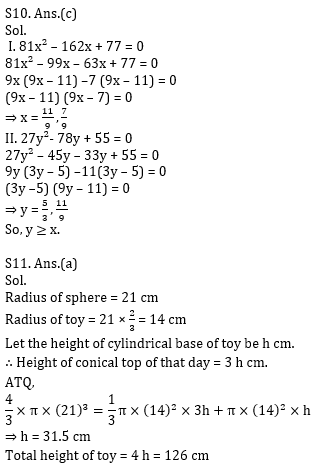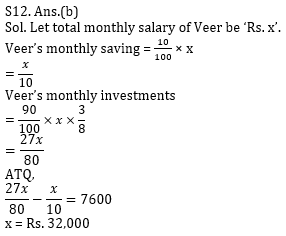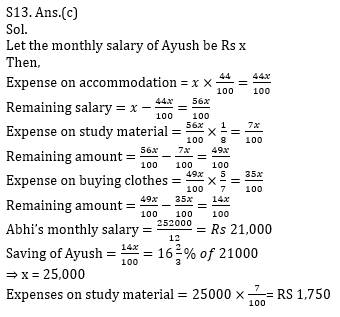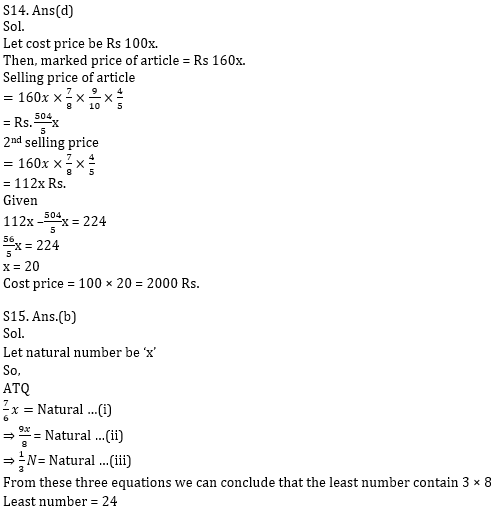Practice with Crash Course and Online Test Series for IBPS Clerk Prelims: